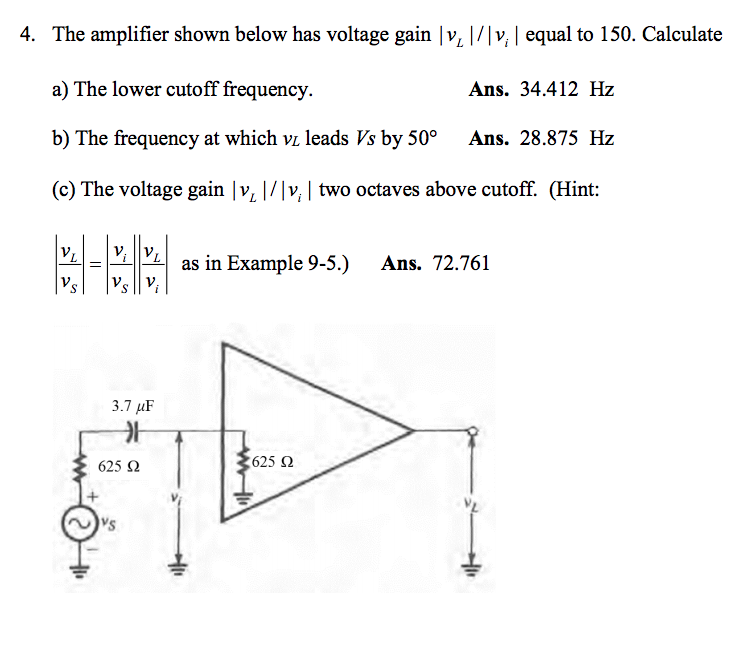# 4. The amplifier shown below has voltage gain v v, equal to 150. Calculatea) The lower cutoff frequency.b) The frequency at which vi leads Vs by 50° Ans. 28.875 Hz(c) The voltage gain |v |/v, two octaves above cutoff. (HintYLLELİİVLİ as in Example 9-5.) Ans. 72.761Ans. 34.412 Hz3.7 μF_가625 2625 Ω

Question
52 views

I got parts a & b right. I just need a clarification how the author got the answer to part c 72.761help_outlineImage Transcriptionclose4. The amplifier shown below has voltage gain v v, equal to 150. Calculate a) The lower cutoff frequency. b) The frequency at which vi leads Vs by 50° Ans. 28.875 Hz (c) The voltage gain |v |/v, two octaves above cutoff. (Hint YLLELİİVLİ as in Example 9-5.) Ans. 72.761 Ans. 34.412 Hz 3.7 μF _가 625 2 625 Ω fullscreen
check_circle

Step 1

From Part (a), the lower cutoff frequency (fL) is 34.412 Hz.

Step 2

Write the expression for required voltage gain as follows:

Step 3

From the amplifie...

### Want to see the full answer?

See Solution

#### Want to see this answer and more?

Solutions are written by subject experts who are available 24/7. Questions are typically answered within 1 hour.*

See Solution
*Response times may vary by subject and question.
Tagged in

### Electrical Engineering